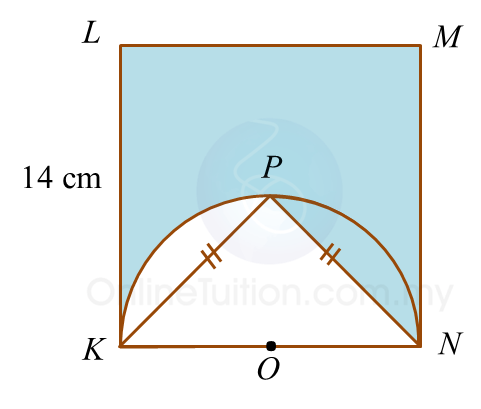# 10.2.1 Circles I, PT3 Focus Practice

10.2.1 Circles I, PT3 Focus Practice

Question 1:
Diagram below shows a circle with centre O.

The radius of the circle is 35 cm.
Calculate the length, in cm, of the major arc AB.

Solution:
Angle of the major arc AB = 360o – 144o= 216o
$\begin{array}{l}\text{Length of major arc}AB\\ =\frac{{216}^{o}}{{360}^{o}}×2\pi r\\ =\frac{{216}^{o}}{{360}^{o}}×2×\frac{22}{7}×35\\ =132\text{cm}\end{array}$

Question 2:
In diagram below, O is the centre of the circle. SPQ and POQ are straight lines.

The length of PO is 8 cm and the length of POQ is 18 cm.
Calculate the length, in cm, of SPT.

Solution
:
Radius = 18 – 8 = 10 cm
PT2 = 102 – 82
= 100 – 64
= 36
PT = 6 cm
Length of SPT = 6 + 6
= 12 cm

Question 3:
Diagram below shows two circles. The bigger circle has a radius of 14 cm with its centre at O.
The smaller circle passes through O and touches the bigger circle.

Calculate the area of the shaded region.

Solution:

Question 4:
Diagram below shows two sectors. ABCD is a quadrant and BED is an arc of a circle with centre C.

Calculate the area of the shaded region, in cm2.

Solution
:

Question 5:
Diagram below shows a square KLMN. KPN is a semicircle with centre O.Calculate the perimeter, in cm, of the shaded region.

Solution
: